# What you need to know about transimpedance amplifiers – part 2

Other Parts Discussed in Post: TINA-TI

In the first installment of this series, I described various factors that affect the loop gain of a transimpedance amplifier (TIA) and demonstrated how to compensate a TIA to achieve a Butterworth (maximally flat) closed-loop response. In this installment, I will show you how to compensate a TIA for an arbitrary phase margin.

The closed-form equations to determine the closed-loop bandwidth and feedback capacitance (CF) for TIA compensation can be quite involved. A simpler approach is to first compensate the TIA for a Butterworth response (Q = 0.707) using the theory presented in part 1 of this series and then, using Figure 1 below, determine the CF to achieve the desired quality factor (Q). Figure 1 also gives the resulting closed-loop bandwidth of the amplifier for the desired Q factor.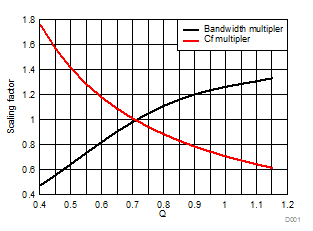Figure 1: Scaling factor for bandwidth (f - 3dB) and CF vs. Q factor

Using the example for a Butterworth response from part 1 of this series, set CF = 0.14pF, which results in f-3dB = 10MHz. To achieve a Q = 0.6, from Figure 1 the resulting CF = 0.14pF x 1.190 = 0.17pF and the resulting f-3dB = 10MHz x 0.82 = 8.2MHz.

For more accurate results, you can download this Excel spreadsheet from the TI E2E™ Community. Figure 2 is a screenshot of two calculators in the spreadsheet. Calculators A and B determine the value of both f-3dB and CF for a Butterworth response and an arbitrary value of Q, respectively.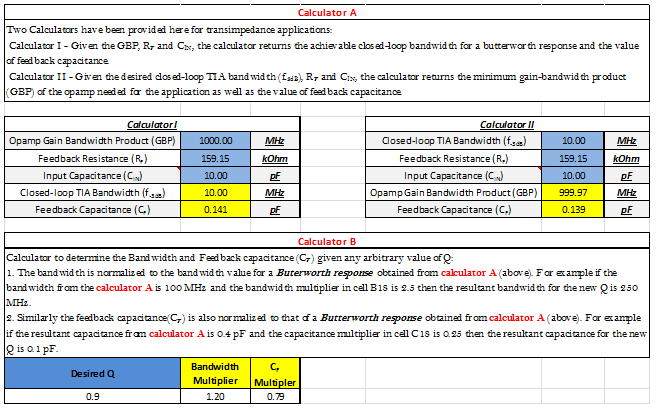Figure 2: Excel calculators to aid in TIA design

The Q of a circuit is directly related to its phase margin (ΦM), which determines the amplifier’s closed-loop frequency response and time-domain pulse response. An amplifier circuit with a low phase margin has a peaked frequency response and significant ringing in the pulse response. Conversely, a circuit with high phase margin has a flat frequency response and little or no overshoot in the pulse response.

Circuits with low phase margin extend the amplifier’s closed-loop bandwidth, while circuits with high phase margin reduce the closed-loop bandwidth. A Butterworth response, which has a Q = 0.707 and a maximally flat frequency response, has a phase margin of 65.5 degrees and about 4.3% overshoot in the pulse response. Figure 3 shows the Q and overshoot as a function of phase margin.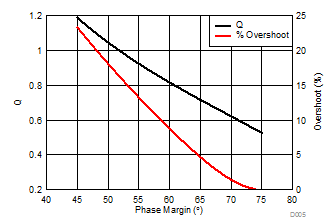Figure 3: Q and overshoot vs. phase margin

To simplify the circuit design, the Excel spreadsheet also has Calculator C, with three separate calculators showing the relationships between Q, overshoot, phase margin and frequency-response peaking.

Calculator C1 gives the phase margin and overshoot for a given value of Q.

Calculator C2 calculates the Q and overshoot for a given value of phase margin. This calculator is useful for achieving a desired frequency-response shape for stability and for maximizing flatness in narrowband applications.

Calculator C3 is useful in time-domain applications when you want to target a certain value of overshoot in the pulse response.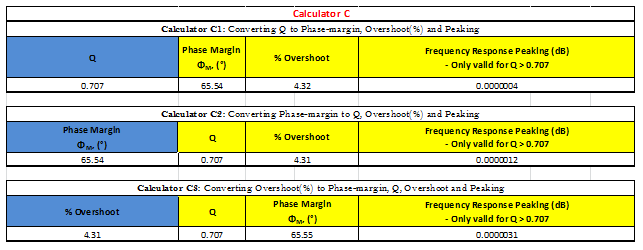Figure 4: Calculator C relating Q, phase margin and overshoot

Using the three different calculators, I calculated the frequency- and time-domain responses for the TIA discussed in part 1 of this series for different values of Q:

• Calculator A produced the CF and f-3dB for a Butterworth response with the specified op amp transimpedance gain and input capacitance.
• Calculator B gave the scaling factor for CF and the change in f-3dB for different values of Q.
• Calculator C predicted the peaking in the frequency response and the overshoot in the pulse response.

The simulated results in Figure 5 match the calculated values very closely.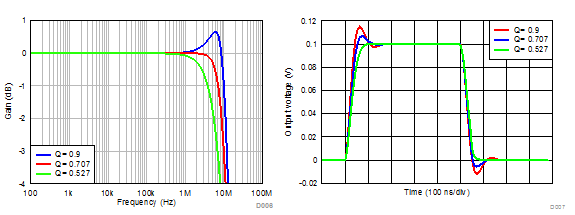Figure 5: Simulated closed-loop frequency and transient responses as a function of Q

You should now be very comfortable with compensating a TIA based on the specific application requirements. The calculators introduced in this post are intended for use with TINA-TI™ software to provide a starting point for TIA design. In the next installment of this series, I will describe the effects of the amplifier’s higher-order open-loop poles on the loop gain of a TIA and introduce the concept of decompensated amplifiers.